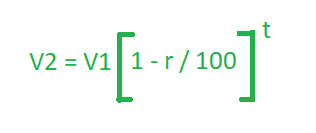# Program to find the Depreciation of Value

The value of any article or item subject to wear and tear, decreases with time. This decrease is called its Depreciation. Given three variable V1, R and T where V1 is the initial value, R is the rate of depreciation and T is the time in years. The task is to find the value of the item after T years.

Examples:

Input: V1 = 200, R = 10, T = 2
Output: 162

Input: V1 = 560, R = 5, T = 3
Output: 480.13

## Recommended: Please try your approach on {IDE} first, before moving on to the solution.

Approach: As in Compound Interest, interest is regularly added to the principal at the end of the agreed intervals of time to generate a new and fresh principal. Similarly, Depreciated value is the decreased value from the amount at the end of agreed intervals of time to generate a new Value.

Thus if V1 is the value at a certain time and R% per annum is the rate (the rate can not be more than 100%) of depreciation per year, then the value V2 at the end of T years is:Below is the implementation of the above approach :

## C++

 `// CPP program to find depreciation of the value ` `// initial value, rate and time are given ` `#include ` `using` `namespace` `std; ` ` `  `// Function to return the depreciation of value ` `float` `Depreciation(``float` `v, ``float` `r, ``float` `t) ` `{ ` ` `  `    ``float` `D = v * ``pow``((1 - r / 100), t); ` ` `  `    ``return` `D; ` `} ` ` `  `// Driver Code ` `int` `main() ` `{ ` `    ``float` `V1 = 200, R = 10, T = 2; ` ` `  `    ``cout << Depreciation(V1, R, T); ` ` `  `    ``return` `0; ` `} `

## Java

 `// Java program to find depreciation of the value ` `// initial value, rate and time are given ` `import` `java.io.*; ` ` `  `class` `GFG ` `{ ` ` `  `// Function to return the depreciation of value ` `static` `float` `Depreciation(``float` `v,  ` `                          ``float` `r, ``float` `t) ` `{ ` `    ``float` `D = (``float``)(v * Math.pow((``1` `- r / ``100``), t)); ` ` `  `    ``return` `D; ` `} ` ` `  `// Driver code  ` `public` `static` `void` `main(String[] args) ` `{ ` `    ``float` `V1 = ``200``, R = ``10``, T = ``2``; ` `     `  `    ``System.out.print(Depreciation(V1, R, T));  ` `}  ` `} ` ` `  `// This code is contributed by anuj_67.. `

## Python3

 `# Python 3 program to find depreciation of the value ` `# initial value, rate and time are given ` `from` `math ``import` `pow` ` `  `# Function to return the depreciation of value ` `def` `Depreciation(v, r, t): ` `    ``D ``=` `v ``*` `pow``((``1` `-` `r ``/` `100``), t) ` ` `  `    ``return` `D ` ` `  `# Driver Code ` `if` `__name__ ``=``=` `'__main__'``: ` `    ``V1 ``=` `200` `    ``R ``=` `10` `    ``T ``=` `2` ` `  `    ``print``(``int``(Depreciation(V1, R, T))) ` ` `  `# This code is contributed by ` `# Surendra_Gangwar `

## C#

 `// C# program to find depreciation of the value ` `// initial value, rate and time are given ` `using` `System; ` ` `  `class` `GFG ` `{ ` ` `  `// Function to return the depreciation of value ` `static` `float` `Depreciation(``float` `v, ``float` `r, ``float` `t) ` `{ ` ` `  `    ``float` `D = (``float``) (v * Math.Pow((1 - r / 100), t)); ` ` `  `    ``return` `D; ` `} ` ` `  `// Driver code  ` `public` `static` `void` `Main() ` `{ ` `    ``float` `V1 = 200, R = 10, T = 2; ` `     `  `    ``Console.WriteLine(Depreciation(V1, R, T));  ` `}  ` `} ` ` `  `// This code is contributed by nidhiva `

Output:

```162
```

My Personal Notes arrow_drop_upCheck out this Author's contributed articles.

If you like GeeksforGeeks and would like to contribute, you can also write an article using contribute.geeksforgeeks.org or mail your article to contribute@geeksforgeeks.org. See your article appearing on the GeeksforGeeks main page and help other Geeks.

Please Improve this article if you find anything incorrect by clicking on the "Improve Article" button below.

Improved By : nidhiva, vt_m, SURENDRA_GANGWAR

Article Tags :
Practice Tags :

Be the First to upvote.

Please write to us at contribute@geeksforgeeks.org to report any issue with the above content.